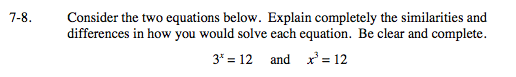Home > INT3 > Chapter 7 > Lesson 7.1.1 > Problem7-8

7-8.
1. Consider the two equations below. Explain completely the similarities and differences in how you would solve each equation. Be clear and complete. Homework Help ✎

2. 3x = 12 and x3 = 12Where is the variable located in each equation? What method should be used to solve each equation?